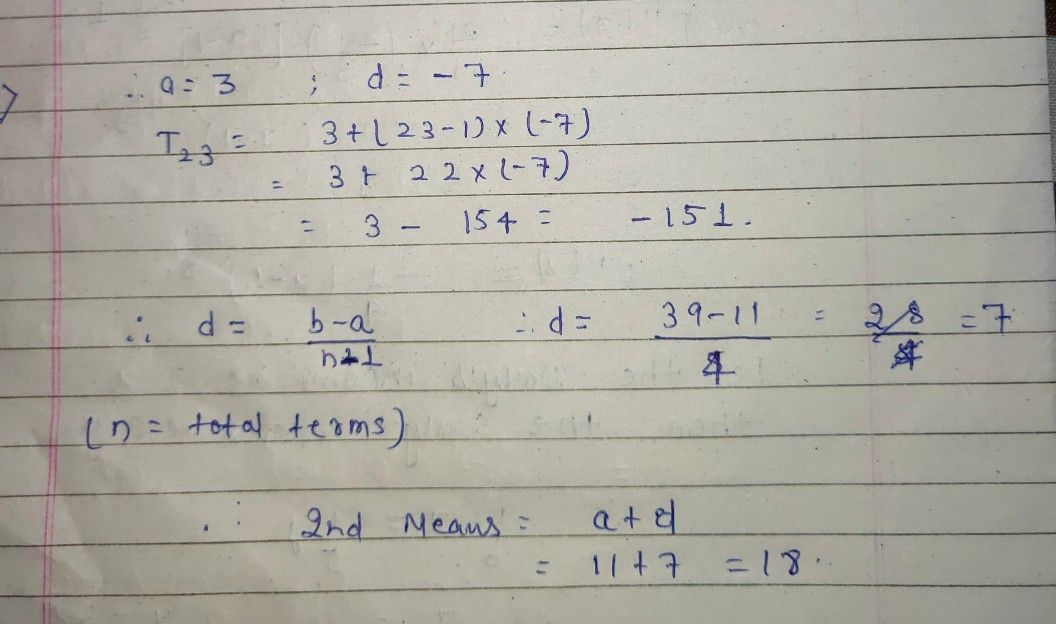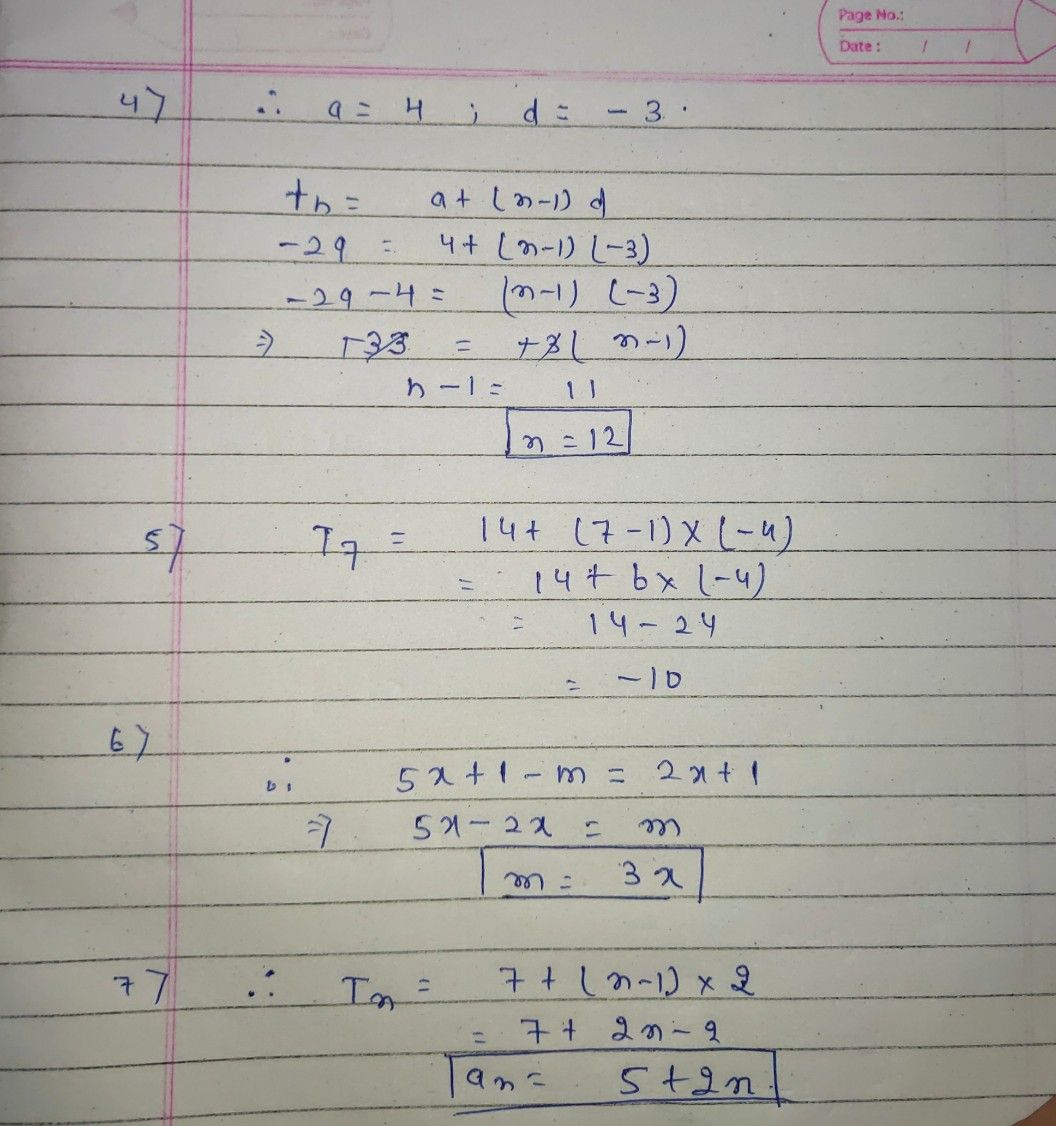Symbol
ProblemC. 6 D. 12 2. The first term of an arithmetic sequence is 3 and the common difference is -7. Find the 23d term. $A-151$ B. - 144 C - 137 $D$ $-130$ 3. If three arithmetic means are inserted between 11 and 39, find the second arithmetic mean. $A1B$ B. 25 C. 32 D. 46 4. Which term of the arithmetic sequence $4,1.-2.5$ $-5....$ $13-293$ A. 9th term B. 10th term C. 11th term D. 12th term 5. What is the 7 term of the arithmetic sequence $14,10.6.2$ 2,....? $A=4$ B. -5 C. -6 $D-10$ 6. $m,5x+1,nf_{0\pi m}$ an arithmetic sequence. If the common difference is $2x41$ what is m equal to? $A7x+3$ B. $5x=2$ C. 5x D. 3x 7. What is the nth term of the arithmetic sequence $7.9.11.13$ ...? $A.a_{n}=3n+4$ $B.a_{n}=4n+3$ C. $a_{n}=n+2$ $D:a_{n}=2n+5$
10th-13th grade
Other
Search count: 128
SolutionQanda teacher - Sahilkhanso 2.. option A 3.. option A4 . option D 5 option D 6. option D 7 option D